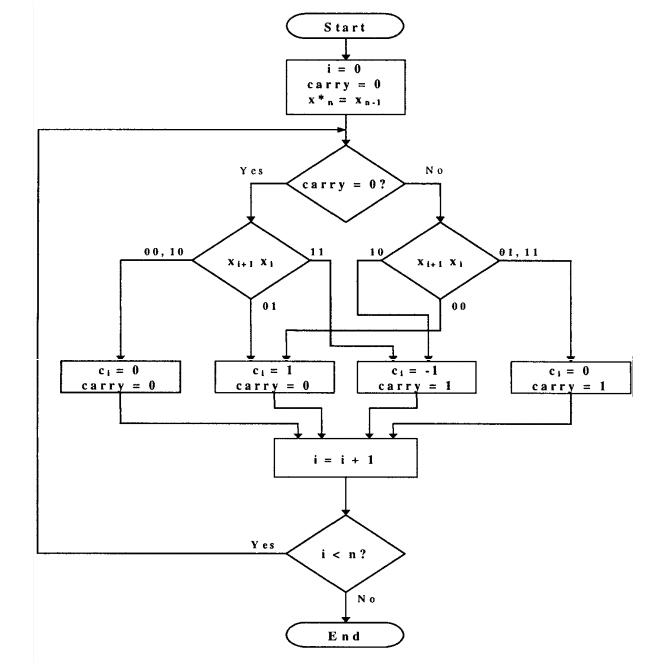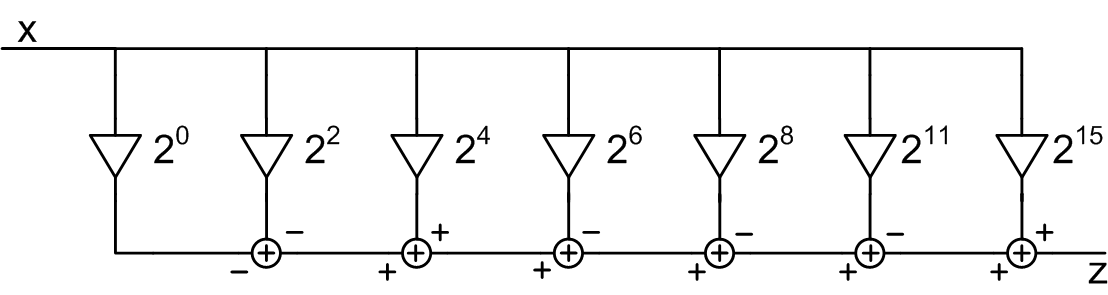Technical Article

# An Introduction to Canonical Signed Digit Representation

July 03, 2017 by Dr. Steve Arar

## CSD is an elegant method to implement digital multipliers in a more efficient way.

CSD is an elegant method to implement digital multipliers in a more efficient way. This article reviews the basics of CSD and its implementation.

Generally‎, ‎digital signal processing algorithms require a large number of multiplications‎. ‎As a simple example‎, ‎consider a FIR filter with 32 taps‎. ‎Utilizing the symmetry in the filter coefficients‎, ‎we need 16 multiplications to produce a single sample of the filter output‎. ‎Depending on the number of bits used to represent the input samples and the filter coefficients‎, ‎these multiplications can easily become a time-consuming process‎. ‎

The design of hardware-efficient multipliers has attracted a lot of attention over the decades ‎and ‎the ‎extensive ‎research ‎has ‎led ‎to a‎ ‎number ‎of ‎solutions. ‎This article reviews the basic concepts of canonical signed digit (CSD) representation. CSD is an interesting solution in implementing efficient multipliers‎, particularly when multiplying an input signal by a constant ‎multiplicand‎‎, ‎e.g‎. ‎when implementing a FIR filter ‎where we face some fixed coefficients‎‎.

### The Main Idea ‎of CSD

Suppose ‎that ‎we ‎want ‎to ‎multiply $$‎x$$‎‎ ‎by ‎the ‎binary ‎number $$‎y=‎‎1‎1‎110$$‎. ‎The ‎simplest ‎way ‎to ‎do ‎this ‎would ‎be ‎based ‎on ‎the ‎following ‎equation:‎

$$‎z‎= ‎2^{4}x+‎‎2^{3}x+2^{2}x+2^{1}x$$‎

Multiplication (and division) by two can be achieved by bitwise shift operations. Hence, the above equation ‎means ‎that ‎we ‎need ‎to ‎add ‎shifted ‎versions ‎of $$x$$‎. The number of shift and addition ‎operations‎ depends on the number of non-zero digits of the multiplicand. In this example, ‎there‎ are ‎four‎ ‎1s, ‎so‎ ‎the ‎multiplication ‎requires ‎four‎ ‎shifts ‎and ‎three ‎additions. ‎If ‎we ‎could ‎represent ‎‎$$y$$ with fewer non-zero digits, then we ‎could‎ reduce the complexity of multiplication. ‎In ‎this ‎particular ‎example, ‎it ‎is‎ ‎actually possible to represent ‎$$y$$ in a more efficient way ‎because $$‎y=30=2^{5}-2^{1}$$. ‎With ‎this ‎representation, ‎called ‎CSD,‎ ‎the ‎above ‎multiplication ‎needs ‎two ‎shifts ‎and ‎only a‎ ‎single ‎subtraction. CSD ‎is a‎ ‎representation ‎in ‎which ‎the ‎number ‎of ‎non-zero ‎digits ‎becomes ‎minimal. To ‎achieve ‎this, ‎CSD ‎uses a‎ ‎ternary ‎number ‎system ‎which ‎uses‎ ‎a‎ ‎new‎ ‎digit, $$‎-1$$, ‎‎‎‎denoted by $$‎\bar{1}$$. For example, the CSD representation of ‎‎$$30‎$$ ‎is ‎‎$$1000\bar{1}0‎$$.‎‎ ‎While ‎the‎ ‎representation ‎in the equation above ‎relies ‎on a‎ ‎summation ‎of ‎powers ‎of ‎two, ‎CSD‎ ‎uses ‎both ‎additions ‎an‎d subtractions.

This provides more flexibility ‎in implementing arithmetic functions‎; however, we ‎will ‎face ‎two ‎challenges: ‎firstly, ‎we‎ ‎have ‎to ‎represent ‎each ‎number ‎with ‎the digit set of $$‎\{ -1, 0, 1 \}$$ instead of the binary representation which has the digit set of $$‎\{ 0, 1 \}$$. ‎This ‎means ‎that ‎two ‎bits ‎are ‎required ‎to ‎store ‎one ‎digit ‎of a ‎CSD number.‎ ‎Secondly, ‎since ‎we ‎are ‎generally ‎using ‎the ‎binary ‎representation, ‎we ‎have ‎to ‎add ‎some ‎circuitry ‎which ‎converts‎ a‎ ‎binary ‎number ‎into ‎CSD ‎before performing the multiplication‎. ‎

‎‎‎As ‎the ‎discussed‎ ‎‎example ‎shows, ‎CSD ‎is ‎particularly ‎helpful ‎in ‎dealing ‎with ‎numbers ‎which ‎have a‎ ‎string ‎of ‎1s. ‎In ‎these ‎cases, ‎CSD ‎replaces ‎several ‎shift ‎and ‎add ‎operations ‎resulting ‎from‎ ‎the ‎string ‎of ‎1s‎ ‎with ‎two ‎shifts ‎and ‎one ‎subtract ‎operation.

### Important Properties of CSD Representation

In a‎ ‎CSD ‎number, ‎two ‎consequtive ‎digits ‎cannot be‎‎‎‎ ‎non-zero, ‎i.e., $$1\bar{1}$$ ‎and ‎$$\bar{1}1$$ ‎are ‎not ‎allowed. ‎‎‎Therefore, the maximum number of non-zero digits for an $$N$$-bit CSD number will be $$\lfloor{\frac{N}{2}‎}\rfloor$$. Even with $$N$$, ‎the ‎largest ‎number ‎that ‎can ‎be ‎represented ‎by ‎an‎ $$N$$-bit CSD is

$$‎2^{N-1}+2^{N-3}+‎\dots+2^{1}‎\approx‎ ‎\frac{2^{N+1}}{3}‎‎$$

Hence, ‎while a‎ ‎four-bit ‎binary ‎number ‎can ‎represent ‎the ‎decimal $$15$$, the largest value represented by a‎ ‎four-bit ‎CSD ‎number ‎is $$10$$.‎‎‎

For ‎an $$N$$-bit CSD number, $$C=\sum_{i=0}^{N-1}c_{i} 2^{i}$$‎, the probability of $$c_{i}$$ being non-zero is given by

$$P(|c_{i}| =1)=\frac{1}{3} +\frac{1}{9N} \left[1-\left(-\frac{1}{2} \right)^N\right]$$

With a relatively large $$N$$, the above probability becomes ‎approximately equal to $$‎\frac{1}{3}$$. However, ‎for a binary $$N$$-bit number, ‎the‎ probability of one particular bit being non-zero is $$\frac{1}{2}$$. This ‎means ‎that ‎CSD ‎reduces ‎the‎ ‎number of non-zero digits and leads to a ‎‎‎‎‎‎‎‎‎‎more ‎e‎‎fficient ‎implementation of multiplication.

While the binary representation uses the concept of 2's complement to code the negative numbers, we only need to invert the sign of digits in a ‎‎CSD number to represent negative numbers. This is possible because the digit set ‎of ‎CSD‎ includes $$-1$$. For ‎example, $$‎-‎30$$ ‎is represented by $$\bar{1}00010$$.‎‎

### The Algorithm to Convert from Binary to CSD

There ‎are a‎ ‎number ‎of ‎algorithms ‎to ‎convert a‎ ‎binary ‎number ‎to ‎CSD. The simple approach suitable for paper-and-pencil conversion from unsigned binary representation to CSD is ‎to ‎search the number from LSB to ‎MSB,‎ find a ‎‎‎string ‎of ‎1‎s ‎followed by a 0‎, ‎e.g. ‎$$0111$$,‎ ‎and ‎replace ‎them ‎with the CSD representation, i.e., $$100\bar{1}$$. We may need to repeat this process several times because there may be other strings of ones, or new strings of ones may be produced as a result of the previous iteration. Let's see some examples to clarify the process:

Example 1: Find the CSD equivalent of $$01011101$$.

In the first iteration of searching from LSB to MSB, we find the string $$0111$$ which can be represented by $$100\bar{1}$$. After replacing this string with its CSD representation, we have $$01100\bar{1}01$$. Now, we again search the obtained number from LSB to MSB to see if there is any other string of ones. We see that a new string is generated due to the first iteration of the process: $$011$$. This can be substituted by $$10\bar{1}$$. Then the CSD equivalent of $$01011101$$ will be $$10\bar{1}00\bar{1}01$$.

Example 2: Find the CSD representation of $$1010111$$.

In the first iteration, we find $$0111$$ and replace it with $$100\bar{1}$$, and we obtain $$101100\bar{1}$$. In the second iteration, we find $$011$$ and replace it with $$10\bar{1}$$. Hence, we have $$110\bar{1}00\bar{1}$$. Now, there is another string $$11$$. To find the CSD equivalent of this iteration, we need to add a $$0$$ to the leftmost position of $$110\bar{1}00\bar{1}$$. Obviously, this will not change the value of this number, but it will allow us to replace $$011$$ with $$10\bar{1}$$ . Finally, we obtain the CSD representation as $$10\bar{1}0\bar{1}00\bar{1}$$.

The‎ formal ‎algorithm‎ ‎flowchart, which is suitable for hardware implementation, is ‎illustrated ‎in ‎the ‎following ‎figure. ‎The algorithm converts from 2's complement to CSD. In Figure 1,‎ $$x_{i}$$ and $$c_{i}$$ denote the input binary number and the output CSD coded number, respectively. ‎The‎ ‎algorithm ‎needs to define an ‎auxiliary‎ ‎signal "carry" ‎and ‎replicate ‎the ‎MSB of the $$n$$-bit binary ‎number, $$x_{n-1}$$‎,‎ ‎at ‎the bit ‎position $$x_{n}$$.### How to Perform Multiplication with CSD?

As discussed above, a CSD multiplier is faster than a conventional binary multiplier. However, before using CSD representation we need to convert ‎the‎ multiplicand from binary to CSD. When one of the multiplicands is fixed, we can easily calculate the CSD equivalent of the coefficient and implement it.‎‎

For example, ‎suppose ‎that ‎we ‎have ‎designed ‎a‎ FIR filter and we need to ‎implement‎ multiplication by a fixed coefficient, say $$0.30410913$$‎. Generally, we apply a scaling factor ‎and ‎implement ‎the ‎rounded ‎coefficient. ‎The ‎scaling ‎factor ‎is ‎chosen based on both the magnitude of the largest coefficient and the number of ‎bits that will be used for representing the coefficients. ‎Suppose ‎that ‎our ‎largest ‎coefficient ‎is‎ $$0.30410913$$ ‎and ‎we ‎are ‎going ‎to ‎represent ‎each ‎coefficient ‎with $$16$$ ‎bits. ‎Then, ‎the ‎scaling ‎factor ‎can ‎be ‎as ‎large ‎as‎ $$\frac{2^{15}-1}{0.3041091‎3‎} \approx 107750$$. ‎Choosing ‎the ‎scaling ‎factor ‎equal ‎to $$‎10^5$$ means we will have to implement the rounded coefficient $$30411$$ which is $$0111011011001011$$. ‎The ‎CSD ‎representation ‎of ‎this ‎coefficient ‎is $$1000\bar{1}00\bar{1}0\bar{1}010\bar{1}0\bar{1}$$. ‎The ‎following ‎figure ‎shows ‎how ‎the ‎multiplication ‎of ‎this ‎CSD ‎number ‎by $$x$$ ‎can ‎be ‎implemented.‎##### Figure 2. A possible ‎implementation ‎for‎ ‎multiplication ‎by‎ $$1000\bar{1}00\bar{1}0\bar{1}010\bar{1}0\bar{1}$$‎.

While ‎multiplication ‎by a‎ ‎constant ‎CSD ‎number ‎is ‎straightforward, ‎multiplication ‎by a‎ ‎varying ‎multiplicand ‎requires ‎some ‎extra ‎circuitry ‎to ‎convert ‎the ‎binar‎y number into CSD representation. We can use a lookup-table based method or ‎directly ‎implement ‎the ‎algorithm ‎shown ‎in ‎Figure ‎1.‎

As a‎ ‎final ‎note, ‎remember ‎that ‎sometimes ‎‎we ‎can ‎even ‎further ‎simplify ‎CSD‎ ‎multiplications. For example, when designing a FIR filter, it is possible to introduce a small error in the magnitude response of the filter and achieve a much more efficient system. Some other designs‎‎ rely on the idea that with different scaling factors, the number of non-zero digits of the CSD representation ‎of ‎a ‎FIR ‎filter‎ ‎varies. ‎Utilizing ‎this ‎property, ‎it ‎is ‎possible ‎to ‎find a‎ ‎better ‎implmentation ‎by‎ examining various scaling factors. This is more interesting than the previous solution because here we are not trading efficiency for accuracy. ‎•MD ZAKIR HUSSAIN November 22, 2017